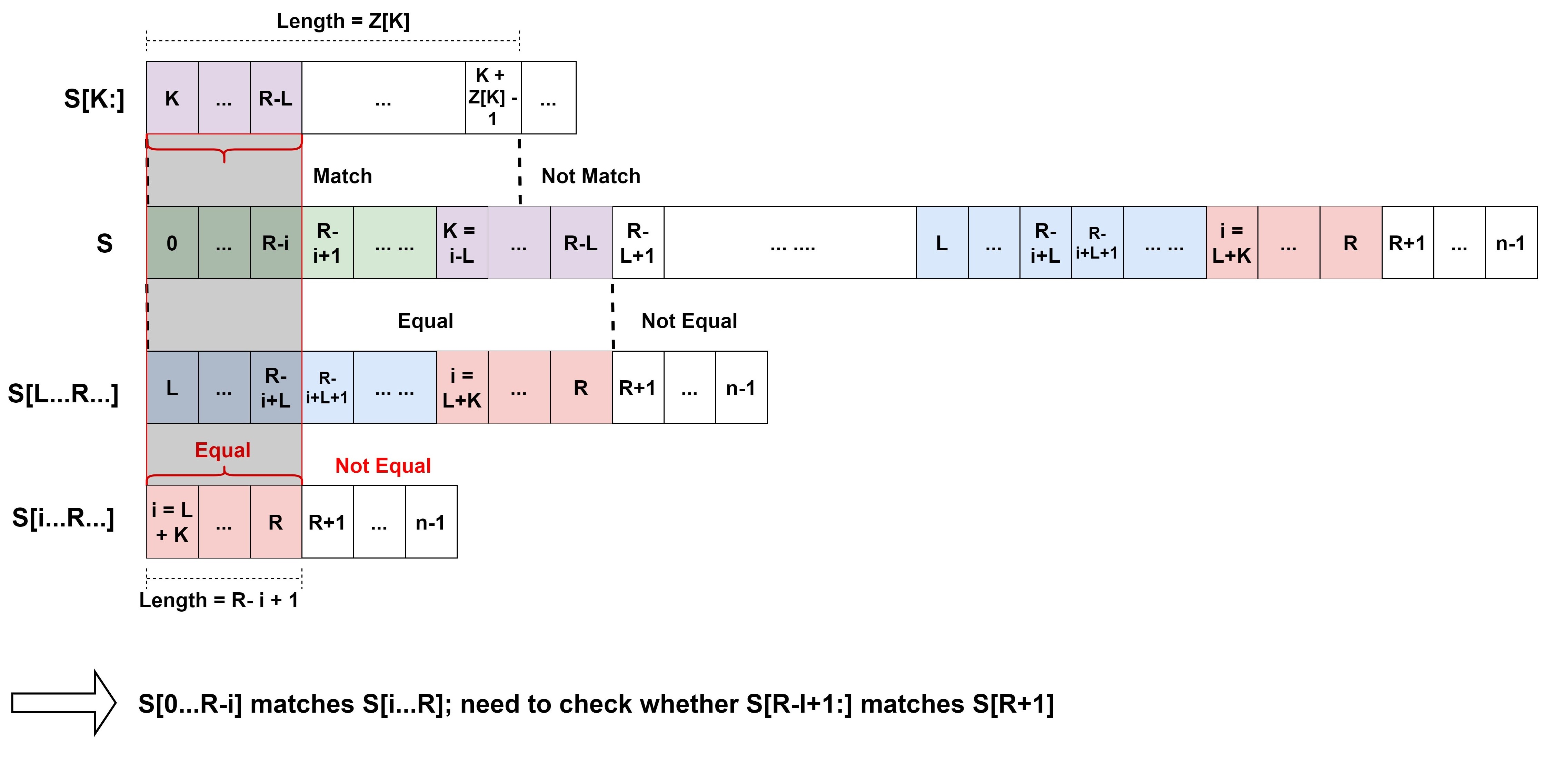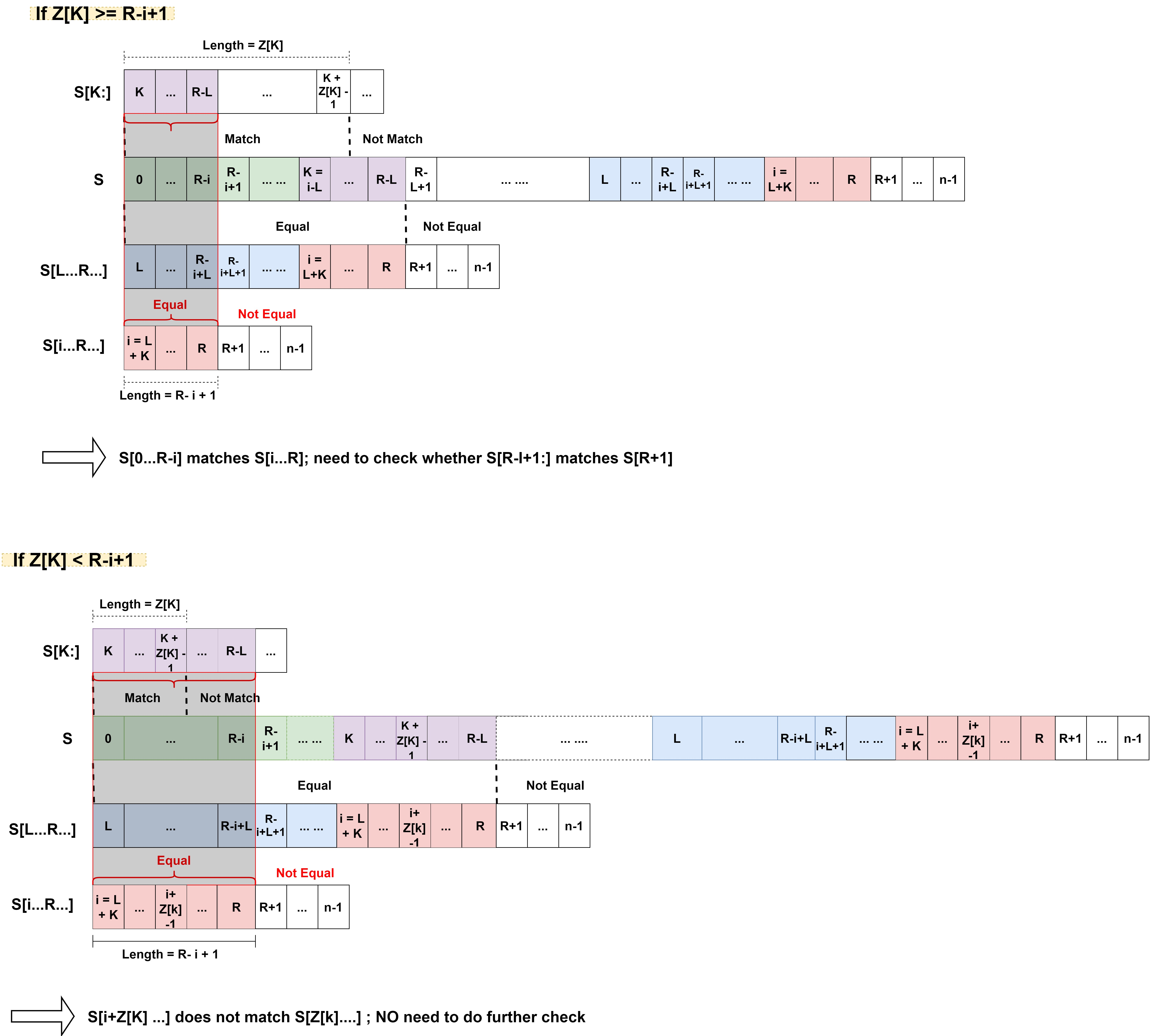Original

Z algorithm - string searching

Assume that we have a string s of length n. Z algorithm runs in linear time O(n) to calculate the lengths of the longest substrings starting from s[i] (i=0, 1...n-1) which is also a prefix of s[0..n-1].

Steps

z is an array that stores the lengths of the common prefixes between each suffix s[i:] and the original string s. Z algorithm loops through i = 1 to n-1 to calculate z[i]. We use L and R (left and right) to keep track of the substring with max R (s[L...R]) which is also the prefix of s.

1. Let L = R = 0, z = n initially.

2. Loop through i = 1 to n-1

1) if i > R:

Use brute force to find common prefix between s and s[i:] and update z[i], L and R.

2) if i <= R:

We've already known that s[L...R] == s[0...R-L]. Therefore, instead of comparing s and s[i:] directly, we can first compare s[L...R] and s[i...R] to decide weather it is neccessary to further compare s[R-i+1:] with s[R+1:] if s[L...R] == s[0..R-L] == s[i...R].

To compare s[L...R] and s[i...R], there is no need to start from scratch. Because the relationship between s[L...R] and s[i...R...] is similar to the relationship between s[0:] and s[i-L:]. We can use the value of z[i-L] that we have already calculated to simplify the calculation of z[i].

PS: The idea here seems like the state transition in dynamic programming to me.

a) If z[i] < R-i+1: z[i] = z[k]

b) If z[i] >= R-i+1: Further compare s[R+1:] and s[R-i+1] until they mismatch. Update L and R.

Illustration:Application of Z algorithm to string searching

Z algorithm can be applied to string searching by connecting two strings with a dummy character.

For example: find "abc" in "adsjdabcsbdbabc"

• The problem will be converted to calculate z[4...] of s and find where z[i] == 3.

Python 3 implementation

def Z(s):
n = len(s)
z = [n] * n
l = r = 0
for i in range(1, n):
if i > r:
l = r = i
while r < n and s[r-i] == s[r]:
r += 1
z[i] = r-l
r -= 1
else:
k = i-l
if z[k] < r-i+1:
z[i] = z[k]
else:
l = i
while r < n and s[r-i] == s[r]:
r += 1
z[i] = r-l
r -= 1
print(z)

References:

 GeeksforGeeks Z algorithm (Linear time pattern searching Algorithm)

• By Yuechun Wang
• Published 2020-09-23
• The materials on this website may be freely copied and distributed for noncommercial personal use only so long as our copyright notice and website address is included.
•